# Test: Power Electronics

## 25 Questions MCQ Test GATE Electrical Engineering (EE) 2023 Mock Test Series | Test: Power Electronics

Description
Attempt Test: Power Electronics | 25 questions in 75 minutes | Mock test for GATE preparation | Free important questions MCQ to study GATE Electrical Engineering (EE) 2023 Mock Test Series for GATE Exam | Download free PDF with solutions
QUESTION: 1

### In the circuit shown, the capacitor has initial voltage V0 with upper plate positive the conduction time for the Diode D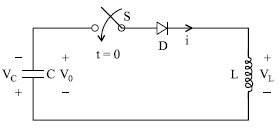Solution: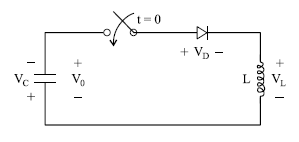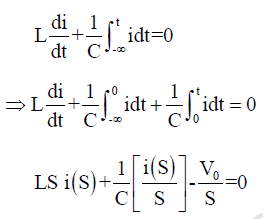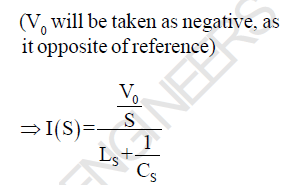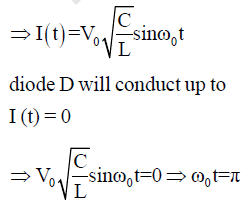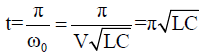QUESTION: 2

### In the above question, the steady state voltage across the capacitor

Solution: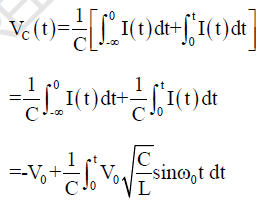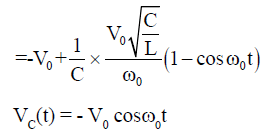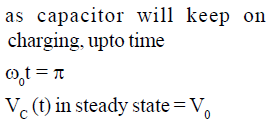QUESTION: 3

### A single phase transformer with secondary voltage 230V, 50Hz, delivers power to load R = 10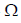through a half wave controlled rectifier circuit. For a firing angle delay at 600, the form factor will be

Solution: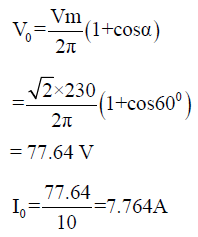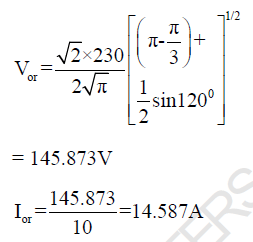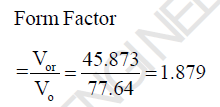QUESTION: 4

In the above question, Voltage ripple Factor will be

Solution: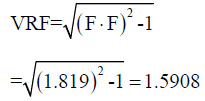QUESTION: 5

The circuit shown in figure is initially relaxed. If the thyristor is turn on at t = 0, what is the conduction time of thyristor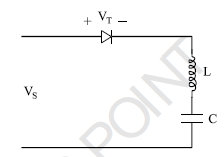Solution:

Apply KVL,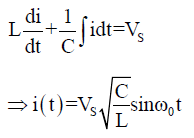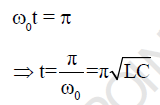QUESTION: 6

In a thyristor, the holding current IH is

Solution:

Latching current is two to three
time the holding current

QUESTION: 7

In the circuit shown below the thyristor is fired at an angle π/4 in every positive half cycle of the input
ac voltage the average power across the load will be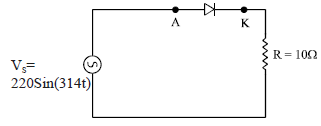Solution: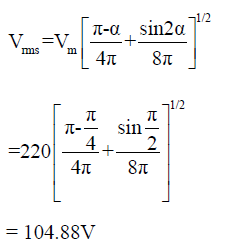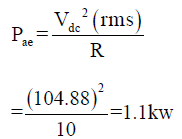QUESTION: 8

A half controlled bridge converter is operating from an r.m.s input voltage of 120V. Neglecting the voltage drops, what are mean load voltage at a firing & delay angle of 00 and 1800 respectively.

Solution:

For half controlled bridge
rectifier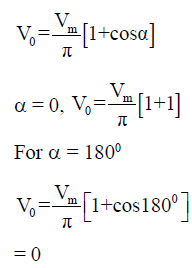QUESTION: 9

In the single phase diode bridge rectifier shown in figure, the load resistor is R = 50The source
voltage is V = 200 sin(ωt), where ω = 2π x 50 rad/s. The power dissipated in the load resistor R is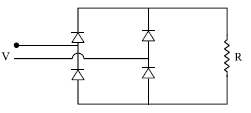Solution: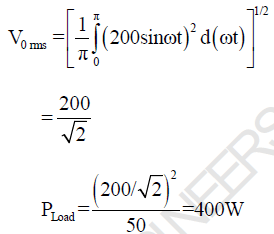QUESTION: 10

A bridge converter is fed from a source VS = Vm sinωt as shown in following figure. What will be the
output voltage for a firing angle of α. Assume continuous conduction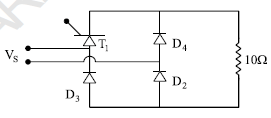Solution: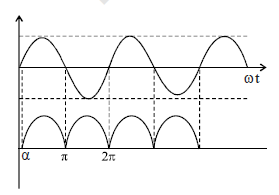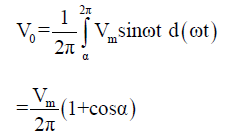QUESTION: 11

A DC battery of 50V is charged through a 10Ω resistor as shown in the figure. Assume that the thyristor is continuously fired. The average value of charging current is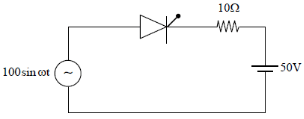Solution: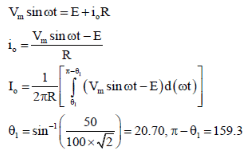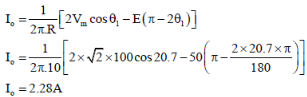QUESTION: 12

In the given figure, if the triggering angle of thyristor is 1200, the extinction angle will be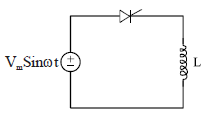Solution: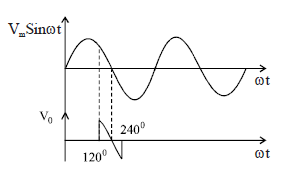as energy is absorbed by the Inductor from 1200 to 1800, as the circuit is resistance less, the same energy will be released by
the inductor in same duration,
hence extinction angle will be β
= 2400

QUESTION: 13

The power electronics converter shown in figure has a single pole double throw switch. The pole P of
the switch is connected alternatively to A and B.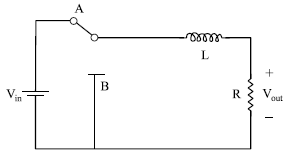The converter shown is a

Solution: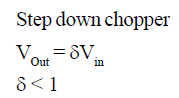QUESTION: 14

In previous question let Vin = 200V and output voltage of 150V load resistance is 10 ohms.
The value of duty cycle is

Solution: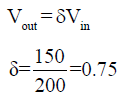QUESTION: 15

In previous question, RMS load current and RMS thyristor current are respectively

Solution: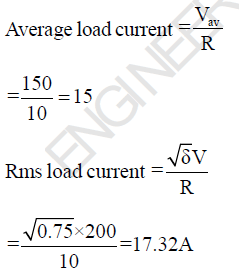QUESTION: 16

What is the value of effective input resistance

Solution: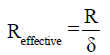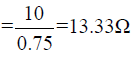QUESTION: 17

The given figure shows a step-down chopper switched at 1 kHz with a duty ratio of 0.5. Assume the load
current is continuous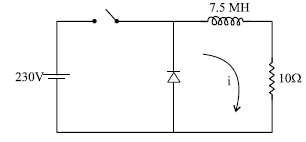The minimum value of instantaneous current is

Solution: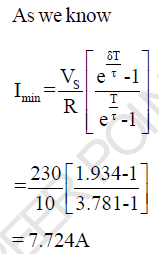QUESTION: 18

In the previous question, the maximum value of instantaneous current is

Solution: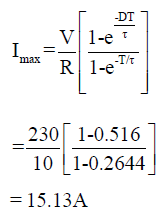QUESTION: 19

A step-down chopper is operated in the continuous conduction mode at a frequency of 4 KHz. If the dc
output and input voltage are 150V and 220V respectively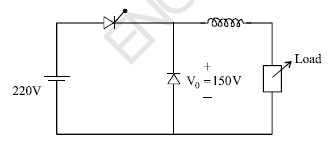the on (Ton) and off period Toff of
the thyristor in each cycle

Solution: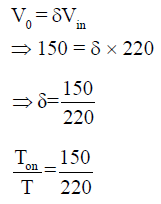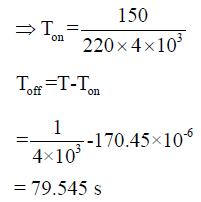QUESTION: 20

The given figure shows a step-down chopper switched at 1 KHz with a duty ratio δ= 0.5, the peak to peak
ripple in load current is close to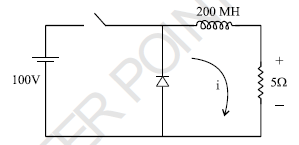Solution: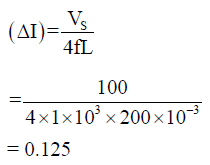QUESTION: 21

The given figure shows a step-down chopper. A free wheeling diode is placed across the load. The load
current varies between the limits of 10A and 12A. The time ratio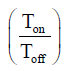would be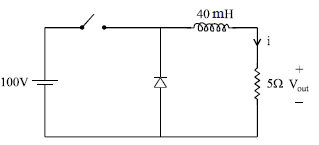Solution: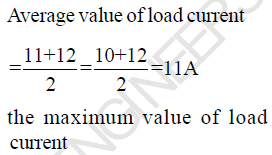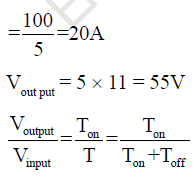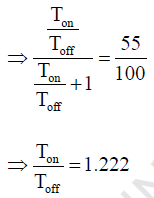QUESTION: 22

A single phase half bridge inverter shown in figure has a resistive load of R = 3and dc input voltage Vdc = 50V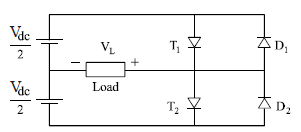the Fundamental component of RMS output voltage and the output power are

Solution: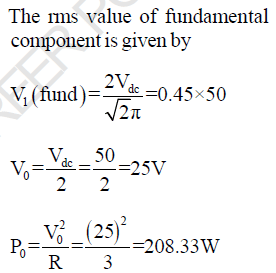QUESTION: 23

In the previous question, the average current through each thyristor will be

Solution: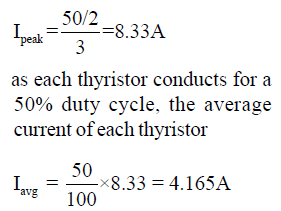QUESTION: 24

A single phase full bridge inverter has square wave output voltage. What is the percentage of fifth
harmonic component in relation to the fundamental component?

Solution: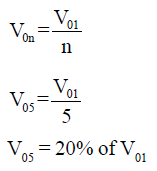QUESTION: 25

A single phase inverter is operated in PWM mode generating a singlepulse of width 2d in centre of each
half cycle as shwon in figure. It is found that output voltage is free from 5th harmonic for pulse width
1440. What will be percentage of 3rd harmonic present in output voltage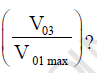Solution: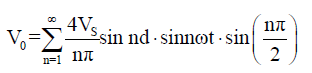as output is free from 5th harmonics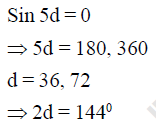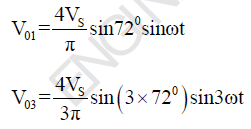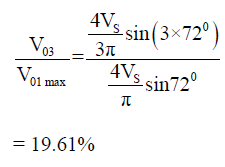Use Code STAYHOME200 and get INR 200 additional OFF Use Coupon Code